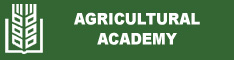Correlation and regression relationships between quantity and quality indicators of forage of Lotus corniculatus L. and Festuca rubra L., treated by bio-fertilizers
Tatyana Bozhanska
Abstract: Correlation and regression dependences between the main chemical indicators of dry biomass of Lotus corniculatus L. (cv. ‚Leo‘) and Festuca rubra L. (cv. ‚Ryder‘), treated with Lumbrical and Lumbrex biofertilizers. The survey was conducted in the experimental field of the Research Institute of Mountain Stockbreeding and Agriculture – Troyan to assess the quality and nutritional value of legume and grass species, under no irrigated conditions and vegetative introduction of biological products. They are a result of the processing of organic waste through the red earthworm (Lumbricus rubellis) and the application of modern biotechnology. High correlation dependence was found between the height of treated grasslands and the crude protein content, hemicellulose and cellulose. The correlation coefficients between the indicators are: r = 0.91, r = 0.64 and r = 0.69 (in bird‘s-foot-trefoil) and r = 0.74, r = 0.96 and r = 0.73 (in red fescue). The imported biological products increased dry matter digestibility for both forage crops. The regression dependence is statistically proven both for the percentage share of leaves (R2 = 0.354) and the mineral content (R2 = 0.777) for bird‘s-foot- trefoil, and the height of the grassland (R2 = 0.897), crude protein (R2 = 0.820), crude fats (R2 = 0.696) and hemicellulose (R2 = 0.955) in the dry forage matter of red fescue. Analyzed data indicates a positive correlation between the percentage share of Festuca rubra L. in the grassland and the values of phosphorus (r = 0.98), mineral substances (r = 0.79) and cellulose (r = 0.59). Regression equations were developed to predict the calcium and phosphorus content by the amount of stem fraction of Lotus corniculatus L. at a high determination coefficient: R2 = 0.939 (Ca) and R2 = 0.907 (P). The correlations between empirically calculated values of nitrogen-free extractable substances were statistically proven with a concentration of: neutral detergent fibers (r = 0.74), acid detergent fibers (r = 0.74), acid detergent lignin (r = 0.59) and partially digestible polyoside cellulose (r = 0.82) in the dry matter composition of bird’s-foot-trefoil.
Keywords: bio-fertilization; correlation and regression dependencies; Festuca rubra L.; Lotus corniculatus L.
Date published: 2021-02-25